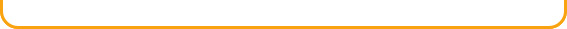Multi-Step Equations

One-Step  Inequalities

Polynomials

Ordered Pairs

Linear Equations

Distance Between Two Points

Intercepts

Slope of a Line

Slope-Intercept Form

Factoring by Grouping

Introduction to  Geometry

Perimeter

Area

Circumference

Volume

Angles

Triangles

Pythagorean Theorem

Trigonometric RatiosSubtraction

Multiplication

Division

Word Problem Solving

Roman Numerals

Exponents

Square Roots

Measurement

Scientific Notation

Decimals and Operations

Introduction to Fractions

Operations with Fractions

Percents, Fractions, and Decimals

Number Relationships

Operations with Signed Numbers

Ratio and Rate

Application of Proportion

Interest Problems

Probability

Graphs

One-Step Equations

Combining Like TermsMath List of TopicsMyStudyBuddy.comTutorials, lessons, quizzes, and other study tools are available for the following topics: